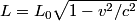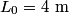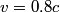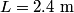## Solution to 1986 Problem 70

 The formula for the relativistic length contraction is\begin{align*}L = L_0 \sqrt{1 - v^2/c^2}\end{align*}Plug in$L_0 = 4 \mbox{ m}$ and$v = 0.8 c$ (the result of the previous problem), to find that$L = 2.4 \mbox{ m}$. Hence answer (A) is correct.